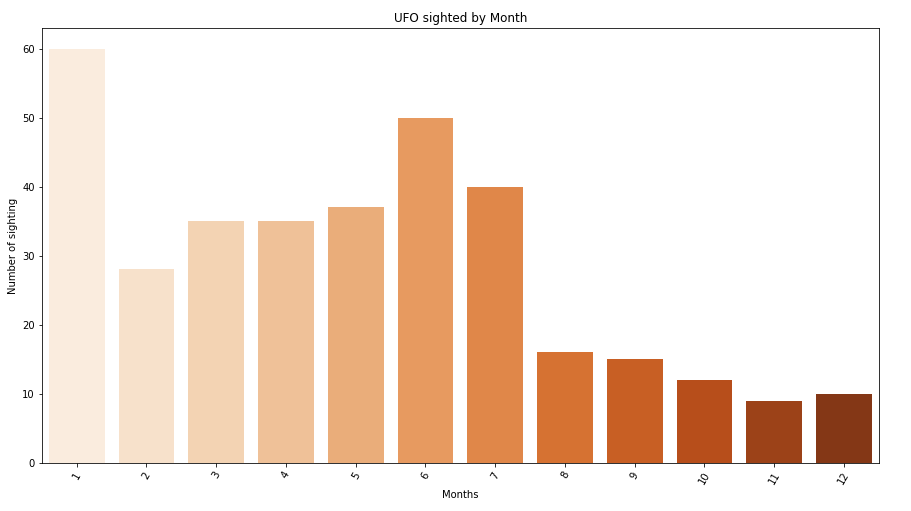﻿ Pandas Datetime: Create a graphical analysis of UFO sighted by month - w3resource

# Pandas Datetime: Create a graphical analysis of UFO sighted by month

## Pandas Datetime: Exercise-21 with Solution

Write a Pandas program to create a graphical analysis of UFO (unidentified flying object) sighted by month.

Sample Solution:

Python Code:

``````import pandas as pd
import matplotlib.pyplot as plt
import seaborn as sns
df['Date_time'] = df['Date_time'].astype('datetime64[ns]')
df["ufo_yr"] = df.Date_time.dt.month
months_data = df.ufo_yr.value_counts()
months_index = months_data.index  # x ticks
months_values = months_data.get_values()
plt.figure(figsize=(15,8))
plt.xticks(rotation = 60)
plt.title('UFO sighted by Month')
plt.xlabel("Months")
plt.ylabel("Number of sighting")
months_plot = sns.barplot(x=months_index[:60],y=months_values[:60], palette = "Oranges")
``````

Sample Output:

``````

Python Code Editor:

Have another way to solve this solution? Contribute your code (and comments) through Disqus.

What is the difficulty level of this exercise?

﻿

## Python: Tips of the Day

F strings:

It is a common practice to add variables inside strings. F strings are by far the coolest way of doing it. To appreciate the f strings more, let's first perform the operation with the format function.

```name = 'Owen'
age = 25
print("{} is {} years old".format(name, age))
```

Output:

```Owen is 25 years old
```

We specify the variables that go inside the curly braces by using the format function at the end. F strings allow for specifying the variables inside the string.

```name = 'Owen'
age = 25
print(f"{name} is {age} years old")
```

Output:

```Owen is 25 years old
```

F strings are easier to follow and type. Moreover, they make the code more readable.

```A, B, C = {2, 4, 6}
print(A, B, C)
A, B, C = ['p', 'q', 'r']
print(A, B, C)
```

Output:

```2 4 6
p q r
```